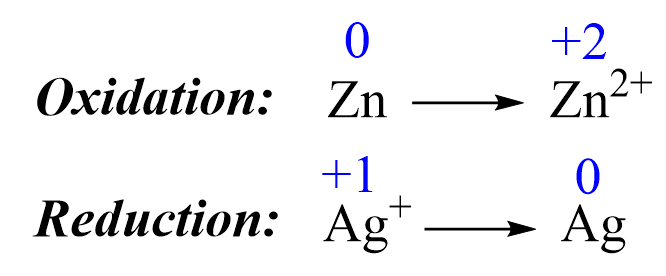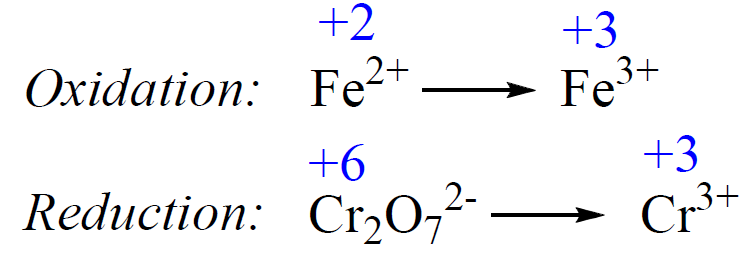## General Chemistry

Redox reactions can be balanced with the half-reaction method. The half-reaction shows the oxidation and reduction processes separately.

For example,

Zn + Ag+   →    Zn2+ + Ag

Follow these steps to balance this reaction:

1) Add the oxidation states and write the half-reactions. The oxidations states are only added to help identify the oxidation and reduction processes:2) Balance the elements. In this case, they are balanced, so proceed to the next step.

3) Balance the charges by adding electrons:

Zn  →  Zn2+ + 2e
1e + Ag+ → Ag

4) Balance the number of electrons by multiplying the equation(s) with a whole number. The first equation has two electrons, and therefore, we multiply the second equation by two:

2 x [1e + Ag+ → Ag] = 2e + 2Ag+ → 2Ag

5) Once everything is balanced, add the half-reactions together and check if all the atoms and charges are balanced again.

Zn  →  Zn2+ + 2e
2e + 2Ag+ → 2Ag

__________________

Zn + 2e + 2Ag+ → 2Ag + Zn2+ + 2e

6) Cancel any species that appear in equal quantitates on both sides of the equation:

Zn + 2e + 2Ag+ → 2Ag + Zn2+ + 2e

Zn + 2Ag+ → 2Ag + Zn2+

# Balancing Redox Equations in Acidic Solution

In the example above, there are only two elements which simplifies balancing the reaction. There is an additional part to balancing redox reactions in acidic or basic conditions which results from the presence of oxygen and hydrogen atoms.

For example,

Fe2+ + Cr2O72- → Fe3+ + Cr3+

Do as we did in the previous example;

1) Add the oxidation states and write the half-reactions:2) Balance the atoms. Add H2O to balance the O atoms, and H+ to balance the H atoms.

The atoms in the first half-reaction are balanced. For the second reaction, we need to equalize the Cr atoms, and add 7 H2O on the right side to balance the oxygen atoms:

Cr2O72- → 2Cr3+ + 7H2O

Now, to balance the H atoms, we need to add 14 H+ on the left side:

14H+ + Cr2O72- → 2Cr3+ + 7H2O

3) Balance the charges by adding electrons.

In the first equation, we add one electron on the right side:

Fe2+ → Fe3++ 1e

In the second equation the charge is zero on the left side (+14 + (-7 x 2) = 0), we have +6 (+3 x 2) on the right side:

14H+ + Cr2O72- → 2Cr3+ + 7H2O

Therefore, add 6eto balance the charges in the second equation:

6e + 14H+ + Cr2O72- → 2Cr3+ + 7H2O

4) Balance the number of electrons by multiplying the equation(s) with a whole number. There are 6 e in the second equation, so multiply the first equation by 6:

6 x [Fe2+ → Fe3++ 1e] = 6Fe2+ → 6Fe3++ 6e

5) Once everything is balanced, add the half-reactions together and cancel the electrons:

6Fe2+ → 6Fe3++ 6e
6e + 14H+ + Cr2O72- → 2Cr3+ + 7H2O

______________________________

6Fe2++ 14H+ + Cr2O72- → 6Fe3+ + 2Cr3+ + 7H2O

Check if everything is balanced. In this case, it is!

# Balancing Redox Equations in Basic Solution

For reactions in basic solutions, we proceed all the way to balancing the equation as if it is in an acidic solution. At the end, for every H+, we add an equal number of OH on both sides of the equation. A pair of H+ and OH ions is then represented as a water molecule.

For example,

MnO4 + I–     MnO2 + I2

1) Write the half-reactions:

MnO4   →     MnO2
I    →     I2

2) Balance the atoms. Add H2O to balance the O atoms, and H+ to balance the H atoms.

The atoms in the first half-reaction are balanced.

For the first reaction, we need to equalize the oxygens, and therefore, we add 2 H2O on the right side:

MnO4  →   MnO2 + 2H2O

And now, add 4 H+ on the left side to balance the hydrogens:

4H+ + MnO4  →   MnO2 + 2H2O

For the second reaction, add 2 in front of I:

2I    →     I2

3) Balance the charges by adding electrons.

To balance the charges in the first equation, we add 3e on the left side because there are three extra positive charges (+4 from the protons and -1 from the permanganate ion):

3e+ 4H+ + MnO4  →   MnO2 + 2H2O

For the second equation, we need to add 2eon the right side:

2I    →     I+ 2e

4) Balance the number of electrons by multiplying the equation with whole numbers. There are 3 electrons in the first equation, and 2 electrons in the second, so ta balance this, we multiply the first equation by 2, and the second equation by 3:

2 x [3e+ 4H+ + MnO4  →   MnO2 + 2H2O] = 6e+ 8H+ + 2MnO4 → 2MnO2 + 4H2O

3 x [2I  →  I+ 2e] = 6I  →  3I+ 6e

5) Once everything is balanced, add the half-reactions together and cancel the electrons:

6e + 8H+ + 2MnO4 → 2MnO2 + 4H2O

6I  →  3I+ 6e

__________________________________________________

8H+ + 2MnO4+ 6I  →  2MnO2 + 4H2O + 3I

6) This is the balanced equation in acidic media, and one more step to make it in a basic media, is to add 8 OH on both sides of the equation to get rid of the 8H+ ions on the left side. This works on the principle of combining H+ and OH ions and representing it as water:

8OH + 8H+ + 2MnO4+ 6I  →  2MnO2 + 4H2O + 3I2 + 8OH

8H2O + 2MnO4+ 6I  →  2MnO2 + 4H2O + 3I2 + 8OH

Notice that we now have 8 H2O on the left side, and only for on the right side. 4 H2O molecules on both sides cancel out, and we are left with the balanced equation in a basic media:

2MnO4+ 6I + 4H2O  →  2MnO2 + 3I2 + 8OH

## To summarize the balancing of redox reactions, remember that:

1) For reactions with only two elements, balance the atoms and then the charges by adding electrons where needed. Once the half-reactions are balanced, add them up and cancel any identical species that appear on both sides of the equation.

2) Balancing redox reactions in acidic media: Follow the same steps and remember, we add H2O to balance the O atoms, and H+ to balance the H atoms.

3) Balancing redox reactions in basic media: Follow the same steps as far balancing in acidic media and, in the end, add as many OH- ions as there are on either side of the equation. Convert an equal number of these ions to water, and cancel the water molecules on both sides of the equation.

Check Also

#### Practice

1.

Balance the following redox reactions occurring in acidic aqueous solution:

a) Al(s) + Fe2+(aq) → Al3+(aq) + Fe(s)

b) SO32(aq) + MnO4(aq) → SO42(aq) + Mn2+(aq)

c) Cr2O72- + C2O22- → Cr3+ + CO2

d) PbO2 + Mn2+ + SO42 → PbSO4 + MnO4

e) MnO4(aq) + H2O2(aq) → Mn2+(aq) + O2(g)

a)
This content is available to registered users only. Click here to Register! By joining Chemistry Steps, you will gain instant access to the answers and solutions for all the Practice Problems and the powerful set of General Chemistry 1 and 2 Summary Study Guides.
b)
This content is available to registered users only. Click here to Register! By joining Chemistry Steps, you will gain instant access to the answers and solutions for all the Practice Problems and the powerful set of General Chemistry 1 and 2 Summary Study Guides.
c)
This content is available to registered users only. Click here to Register! By joining Chemistry Steps, you will gain instant access to the answers and solutions for all the Practice Problems and the powerful set of General Chemistry 1 and 2 Summary Study Guides.
d)
This content is available to registered users only. Click here to Register! By joining Chemistry Steps, you will gain instant access to the answers and solutions for all the Practice Problems and the powerful set of General Chemistry 1 and 2 Summary Study Guides.
e)
This content is available to registered users only. Click here to Register! By joining Chemistry Steps, you will gain instant access to the answers and solutions for all the Practice Problems and the powerful set of General Chemistry 1 and 2 Summary Study Guides.
Solution
This content is available to registered users only. Click here to Register! By joining Chemistry Steps, you will gain instant access to the answers and solutions for all the Practice Problems and the powerful set of General Chemistry 1 and 2 Summary Study Guides.
2.

Balance the following redox reactions occurring in basic aqueous solution:

a) Mn2+ + ClO3 → MnO2 + ClO2

b) MnO4(aq) + Br(aq) → MnO2 + BrO3(aq)

c) MnO4(aq)  + CN(aq) → CNO-(aq)  + MnO2(s)

d) H2O2(aq) + ClO2(aq) → ClO2(aq) + O2(g)

e) MnO4(aq) + Fe(OH)2(s) → MnO2(s) + Fe(OH)3(s)

a)
This content is available to registered users only. Click here to Register! By joining Chemistry Steps, you will gain instant access to the answers and solutions for all the Practice Problems and the powerful set of General Chemistry 1 and 2 Summary Study Guides.
b)
This content is available to registered users only. Click here to Register! By joining Chemistry Steps, you will gain instant access to the answers and solutions for all the Practice Problems and the powerful set of General Chemistry 1 and 2 Summary Study Guides.
c)
This content is available to registered users only. Click here to Register! By joining Chemistry Steps, you will gain instant access to the answers and solutions for all the Practice Problems and the powerful set of General Chemistry 1 and 2 Summary Study Guides.
d)
This content is available to registered users only. Click here to Register! By joining Chemistry Steps, you will gain instant access to the answers and solutions for all the Practice Problems and the powerful set of General Chemistry 1 and 2 Summary Study Guides.
e)
This content is available to registered users only. Click here to Register! By joining Chemistry Steps, you will gain instant access to the answers and solutions for all the Practice Problems and the powerful set of General Chemistry 1 and 2 Summary Study Guides.
Solution
This content is available to registered users only. Click here to Register! By joining Chemistry Steps, you will gain instant access to the answers and solutions for all the Practice Problems and the powerful set of General Chemistry 1 and 2 Summary Study Guides.

### 3 thoughts on “Balancing Redox Reactions”

1.2.•